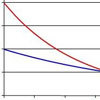#### You may also like### PDF

Given a probability density function find the mean, median and mode of the distribution.### Scale Invariance

By exploring the concept of scale invariance, find the probability that a random piece of real data begins with a 1.### Into the Exponential Distribution

Get into the exponential distribution through an exploration of its pdf.

# PCDF

##### Age 16 to 18 Challenge Level:

James from the MacMillan Academy was the first to crack this problem - well done James!

Steve says

The essence of this problem lies in the fact that a cdf $F(x)$ is non-decreasing and satisfies $0\leq F(x) \leq 1$, whereas a pdf is a non-negative function which integrates to 1 between $-\infty$ and $\infty$. The areas under the curves are the key points to consider. A cdf $F(x)$ can either reach the value $1$ for a finite value of $x_1$ or tend to $1$ as $x\to \infty$. In the first case, provided that the area under the cdf to the left of $x_1$ is 1 then this will work as a pdf for a random variable which is $0$ if $x> x_1$. In the second case, the area diverges and, therefore, cannot be used as a pdf.

To get the curves to match exactly for values of $x< x_1$ we will need to solve the equation $F'(x) = f(x)$.

Here is James's solution

Part 1

A CDF is always an increasing function which goes to $0$ as $X$ goes to $-\infty$ and 1 as x goes to $\infty$ and a pdf is a function that is never negative and has an area under it of 1.

The CDF can reach 1 at any point; let us only consider ones that reach it at 0 as all others either can be generalised from these by moving them left or right i.e. considering $F(x-a)$ where $a$ is the point where it reaches one, or they don't reach 1 until infinity which I shall deal with later.

As the PDF can do what it likes when $x$ is positive (so long as it stays non-negative and doesn't let the area exceed 1) it can make up difference between the area to the left of x=0 and 1 and so the area to the left of x=0 need only be between 0 and 1. So the only rules controlling the CDF are that to the left of where it reaches F(x)=1 the area underneath it must be less than or equal to 1 which is true for any of a multitude of CDF's

Regarding the ones that don't reach 1 until $\infty$ these would have to be a solution to PDF=CDF for $x< \infty$, consider any finite value of $x$ if $F(x)> 0$. At that point then it must be that value or greater until $x=\infty$ giving area under it $\geq F(x)\times \infty$ which is absurd and definitely larger than 1 unless $F(x)$ is infinitesimal for all $x$ in which case $F(x)$ wouldn't be tending to 1 and so such a CDF cannot possibly be a PDF.

Part 2
The integral of a CDF from $-\infty$ to $\infty$ is always $\infty$. Thus a CDF cannot be used as a pdf.

Part 2

As $f(x)=F'(x)$ for $f(x)=F(x)$ we would need $F(x)=F'(x)$. Thus, writing $F(x)=y$, the CDF must be a solution to the differential equation

$$\frac{dy}{dx}=y$$
This gives
$$\frac{1}{y}\frac{dy}{dx}=1$$
Integrating gives
$$\int \frac{1}{y}dy = \int 1 dx$$
Thus $y=f(x)=e^{x+c}$.

Putting in the boundary conditions that $F(0)=1$ give the function $f(x) = e^x$.